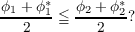#### Vol. 31, No. 3, 1969

 Download this articleFor screen For printingRecent Issues Vol. 325: 1  2 Vol. 324: 1  2 Vol. 323: 1  2 Vol. 322: 1  2 Vol. 321: 1  2 Vol. 320: 1  2 Vol. 319: 1  2 Vol. 318: 1  2Online Archive Volume: Issue:The Journal Subscriptions Editorial Board Officers Contacts Submission Guidelines Submission Form Policies for Authors ISSN: 1945-5844 (e-only) ISSN: 0030-8730 (print) Special Issues Author Index To Appear Other MSP Journals
The average of a gauge

### Charles D. Masiello

Vol. 31 (1969), No. 3, 733–743
##### Abstract

The setting for the discussion is a real linear space with an inner product (x,y). It is assumed that is complete with respect to the norm generated by this inner product. Clearly the gauges on and the bodies (closed, bounded, absolutely convex sets containing the origin 𝜃 as an interior point) generate each other. The norm e(x) = (x,x)is a special gauge; it is customary to write xinstead of e(x). In general, a gauge will be denoted ϕ(x), or briefly by ϕ; the symbol ϕ represents the conjugate of ϕ.

PROBLEM. Consider two gauges ϕ1 and ϕ2 such that e ϕ1 ϕ2. Under what conditions may one conclude that(*)

That such an order relation may exist is suggested by the fact that for any gauge ϕ, its “average” (ϕ + ϕ)2 is well behaving with respect to e, being always e. Moreover, the average of the average is a better approximation to e, and so on. Indeed, it is known that the sequence of successive averages converges decreasingly to e.

SOLUTION. With each gauge ϕ (body K) we associate its spread S(x). The last concept is a very natural one and is defined as follows: For 𝜃x ∈ℒ, consider the line joining 𝜃 and x. Let ω(x) represent the (width) distance between the two support hyperplanes of K orthogonal to that line, while δ(x) represents the (diameter) length of the chord of K lying on that line. We then define Sϕ(x) = SK(x) = ω(x) δ(x). Clearly, S(x) = S(λx) for λ0. It turns out that for any pair of gauges ϕ1 and ϕ2 such that e ϕ1 ϕ2, the relation Sϕ1 Sϕ2 implies inequality () and also ϕ1ϕ1ϕ2ϕ2.

Primary: 46.15
Secondary: 52.00
##### Milestones